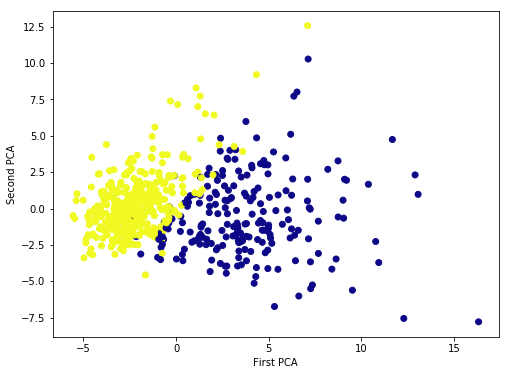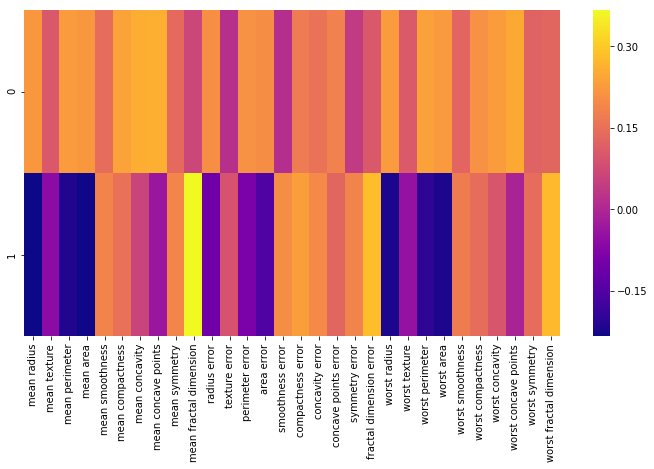# Introduction to Principal Component Analysis

Principal Component Analysis (PCA) is an unsupervised statistical technique used to examine the interrelations among a set of variables in order to identify the underlying structure of those variables. It is also known as a general factor analysis. Factor analysis determines several orthogonal lines of best fit to the data set. Orthogonal means “at right angles”, where the lines are perpendicular to each other in n-dimensional space. n-Dimensional space is the variable sample space which means that a data set with 4 variables will have a 4-dimensional sample space.

Components are a linear transformation that chooses a variable system for the data set such that the greatest variance of the data set comes to lie on the first axis, the second greatest variance on the second axis and so on. This process allows us to reduce the number of variables used in an analysis. As shown below, the normal line of best fit “explains” 70% of the variation whereas the orthogonal line “explains” 28% of the variation. 2% of the variation remains unexplained. Note that components are uncorrelated, since in the sample space they are orthogonal to each other. If we use this technique on a data set with a large number of variables, we can compress the amount of explained variation to just a few components. The most challenging part of PCA is interpreting the components.# How to perform PCA – Python

PCA is just a transformation of your data and attempts to find out what features explain the most variance in your data. We will now walk through the cancer set with PCA.

# 1. Preparation

In :
```import numpy as np
import pandas as pd
import matplotlib.pyplot as plt
import seaborn as sns
%matplotlib inline
```
In :
```from sklearn.datasets import load_breast_cancer
```
In :
```cancer = load_breast_cancer()
```
In :
```cancer.keys() # keys instead of columns because cancer is a dictionary
```
Out:
`dict_keys(['data', 'target', 'target_names', 'DESCR', 'feature_names'])`
In :
```#print(cancer['DESCR'])
```
In :
```df = pd.DataFrame(cancer['data'],columns=cancer['feature_names'])
```
Out:
mean radius mean texture mean perimeter mean area mean smoothness mean compactness mean concavity mean concave points mean symmetry mean fractal dimension worst radius worst texture worst perimeter worst area worst smoothness worst compactness worst concavity worst concave points worst symmetry worst fractal dimension
0 17.99 10.38 122.80 1001.0 0.11840 0.27760 0.3001 0.14710 0.2419 0.07871 25.38 17.33 184.60 2019.0 0.1622 0.6656 0.7119 0.2654 0.4601 0.11890
1 20.57 17.77 132.90 1326.0 0.08474 0.07864 0.0869 0.07017 0.1812 0.05667 24.99 23.41 158.80 1956.0 0.1238 0.1866 0.2416 0.1860 0.2750 0.08902
2 19.69 21.25 130.00 1203.0 0.10960 0.15990 0.1974 0.12790 0.2069 0.05999 23.57 25.53 152.50 1709.0 0.1444 0.4245 0.4504 0.2430 0.3613 0.08758
3 11.42 20.38 77.58 386.1 0.14250 0.28390 0.2414 0.10520 0.2597 0.09744 14.91 26.50 98.87 567.7 0.2098 0.8663 0.6869 0.2575 0.6638 0.17300
4 20.29 14.34 135.10 1297.0 0.10030 0.13280 0.1980 0.10430 0.1809 0.05883 22.54 16.67 152.20 1575.0 0.1374 0.2050 0.4000 0.1625 0.2364 0.07678

5 rows × 30 columns

# 2. PCA Visualization

As we’ve noticed before it is difficult to visualize high dimensional data so we can use PCA to find the first two principal components, and visualize the data in this new, two-dimensional space, with a single scatter-plot. Before we do this though, we’ll need to scale our data so that each feature has a single unit variance.

### Standardising data

In :
```from sklearn.preprocessing import StandardScaler
```
In :
```scaler = StandardScaler()
```
In :
```scaler.fit(df)
```
Out:
`StandardScaler(copy=True, with_mean=True, with_std=True)`
In :
```scaled_data = scaler.transform(df)
```

### Implementing PCA

In :
```from sklearn.decomposition import PCA
```
In :
```pca = PCA(n_components=2)
```
In :
```pca.fit(scaled_data)
```
Out:
```PCA(copy=True, iterated_power='auto', n_components=2, random_state=None,
svd_solver='auto', tol=0.0, whiten=False)```
In :
```x_pca = pca.transform(scaled_data)
```
In :
```scaled_data.shape
```
Out:
`(569, 30)`
In :
```x_pca.shape
```
Out:
`(569, 2)`
In :
```plt.figure(figsize=(8,6))
plt.scatter(x_pca[:,0],x_pca[:,1],c=cancer['target'],cmap='plasma')
plt.xlabel('First PCA')
plt.ylabel('Second PCA')
```
Out:
`Text(0,0.5,'Second PCA')`Clearly by using these two components we can easily separate these two classes.

### Interpreting the components

Unfortunately, with this great power of dimensionality reduction, comes the cost of being able to easily understand what these components represent.

The components correspond to combinations of the original features, the components themselves are stored as an attribute of the fitted PCA object:

In :
```pca.components_
```
Out:
```array([[ 0.21890244,  0.10372458,  0.22753729,  0.22099499,  0.14258969,
0.23928535,  0.25840048,  0.26085376,  0.13816696,  0.06436335,
0.20597878,  0.01742803,  0.21132592,  0.20286964,  0.01453145,
0.17039345,  0.15358979,  0.1834174 ,  0.04249842,  0.10256832,
0.22799663,  0.10446933,  0.23663968,  0.22487053,  0.12795256,
0.21009588,  0.22876753,  0.25088597,  0.12290456,  0.13178394],
[-0.23385713, -0.05970609, -0.21518136, -0.23107671,  0.18611302,
0.15189161,  0.06016536, -0.0347675 ,  0.19034877,  0.36657547,
-0.10555215,  0.08997968, -0.08945723, -0.15229263,  0.20443045,
0.2327159 ,  0.19720728,  0.13032156,  0.183848  ,  0.28009203,
-0.21986638, -0.0454673 , -0.19987843, -0.21935186,  0.17230435,
0.14359317,  0.09796411, -0.00825724,  0.14188335,  0.27533947]])```
In :
```df_comp = pd.DataFrame(pca.components_,columns=cancer['feature_names'])
```
In :
```plt.figure(figsize=(12,6))
sns.heatmap(df_comp,cmap='plasma')
```
Out:
`<matplotlib.axes._subplots.AxesSubplot at 0x1a1dabe240>`